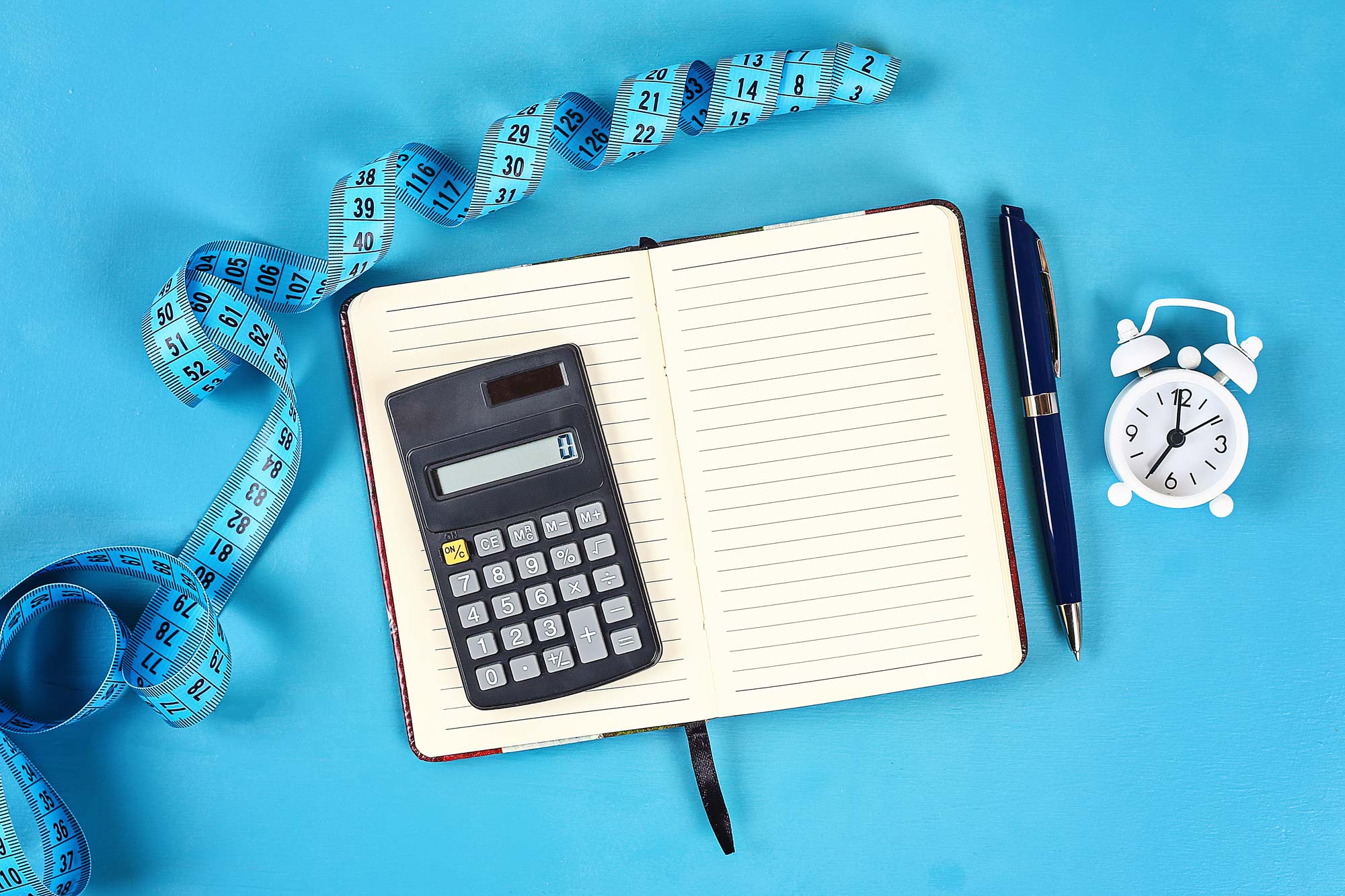Select Page

5,213 total views,  3 views today

# Guide On Paper Weight Calculation Formula

by | Mar 30, 2020

Paper Weight Calculation Formulas

Hi everyone,

This is a simple guide on weight calculation while ordering material, mainly paper.

Have noticed that many a time there is doubt in the weight (KG).

Paper Weight Calculation Formula is

Length(cm)/100 x Width(cm)/100 x GSM/1000 x no. of sheets = Total Kilograms (KG) required.

The reason we divide Length and Width by 100 is to convert the Centimeter to Meter.
(for reference – 100 centimeter = 1 meter)

The reason we divide GSM by 1000 is to convert Grams per sq meter to Kilograms per sq meter.
(for reference – 1000 grams = 1 kilogram)
GSM/1000 = KGSM

Let us take an example for easy understanding

Length = 100 cm
Width = 80 cm
GSM = 300
No. of sheets = 5000

L/100 x W/100 x GSM/1000 x No. of sheets = Total KG
100/100 x 80/100 x 300/1000 x 5000 = 1200 KG

-x-x-x-x-
If calculating for plastic, the material is ordered in KG however it is specified in microns.
we have to do the same as above but multiply with the density of a material.

PP’s density is 0.92.

L/100 x W/100 x GSM/1000 x No. of sheets x density = Total KG
100/100 x 80/100 x 300/1000 x 5000 x 0.92 = 1104 KG

-x-x-x-x-
If calculating for fluting used in corrugation jobs.
The paper used in fluting is more than the paper used in the top and back layers.

The take-up factor for E flute is 1.4

L/100 x W/100 x GSM/1000 x No. of sheets x take up factor = Total KG
100/100 x 80/100 x 300/1000 x 5000 x 1.4 = 1680 KG

-x-x-x-x-
I hope this is clear! If any doubts please write to me 🙂

# Guide On Paper Weight Calculation Formula

by | Mar 30, 2020Hi everyone,
This is a simple guide on weight calculation while ordering material, mainly paper.
Have noticed that many a time there is doubt in the weight (KG).

Formula is
Length(cm)/100 x Width(cm)/100 x GSM/1000 x no. of sheets = Total Kilograms (KG) required.

The reason we divide Length and Width by 100 is to convert the Centimeter to Meter.
(for reference – 100 centimeter = 1 meter)

The reason we divide GSM by 1000 is to convert Grams per sq meter to Kilograms per sq meter.
(for reference – 1000 grams = 1 kilogram)
GSM/1000 = KGSM

Let us take an example for easy understanding

Length = 100 cm
Width = 80 cm
GSM = 300
No. of sheets = 5000

L/100 x W/100 x GSM/1000 x No. of sheets = Total KG
100/100 x 80/100 x 300/1000 x 5000 = 1200 KG

-x-x-x-x-
If calculating for plastic, the material is ordered in KG however it is specified in microns.
we have to do the same as above but multiply with the density of a material.

PP’s density is 0.92.

L/100 x W/100 x GSM/1000 x No. of sheets x density = Total KG
100/100 x 80/100 x 300/1000 x 5000 x 0.92 = 1104 KG

-x-x-x-x-
If calculating for fluting used in corrugation jobs.
the paper used in fluting is more than the paper used in the top and back layers.

The take-up factor for E flute is 1.4

L/100 x W/100 x GSM/1000 x No. of sheets x take up factor = Total KG
100/100 x 80/100 x 300/1000 x 5000 x 1.4 = 1680 KG

-x-x-x-x-
I hope this is clear! If any doubts please write to me 🙂

#### Categories